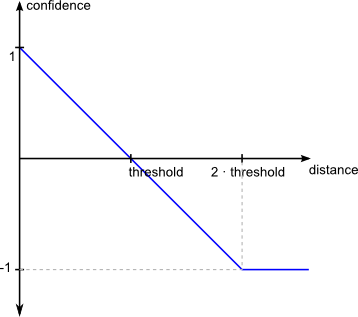# Rule Operators¤

## Introduction¤

Transformation rules are trees that consist of two types of operators:

• Path Operator: Retrieves all values of a specific property path of each entity, such as its label property. The purpose of the path operator is to enable the access of values from the dataset.
• Transformation Operator: Transforms the values of path or transformation operators according to a specific data transformation function.

• Comparison Operator: Evaluates the similarity between two entities based on the values that are returned by two path or transformation operators by applying a distance measure and a distance threshold. Examples of distance measures include Levenshtein, Jaccard, or geographic distance.
• Aggregation Operator: Due to the fact that, in most cases, the similarity of two entities cannot be determined by evaluating a single comparison, an aggregation operator combines the similarity scores from multiple comparison or aggregation operators into a single score according to a specific aggregation function. Examples of common aggregation functions include the weighted average or yielding the minimum score of all operators.

## Path Operator¤

A path operator retrieves all values, which are connected to the entities by a specific path. Every path statement consists of a series of path elements. If a path cannot be resolved due to a missing property or a too restrictive filter, an empty result set is returned.

The following operators can be used to traverse the dataset:

Operator Example Description
`/<property>` `/dbpedia:director/rdfs:label` Moves forward from a subject resource through an operator property to its value(s).
`\<property>` `\dbpedia:artist` Moves backward from a subject resource through an operator property to its value(s).
`[<property> <comp_operator> value]` `/dbpedia:work[rdf:type = dbpedia:Album]` Filters the currently selected values using a filter expression. `comp_operator` may be one of `>`, `<`, `>=`, `<=`, `=`, `!=`
`[@lang = 'lang']` `/rdfs:label[@lang = 'en']` Filter literal values by their language.

## Transformation Operator¤

As different datasets usually use different data formats, a transformation can be used to normalize the values prior to comparison.

Examples of transformation functions include case normalization, tokenization or concatenation of values from multiple operators. Multiple transformation operators can be nested in order to apply a chain of transformations.

## Comparison Operator¤

A comparison operator evaluates two inputs and computes the similarity based on a user-defined distance measure and a user-defined threshold.

The distance measure always outputs 0 for a perfect match, and a higher value for an imperfect match. Only distance values between 0 and the threshold will result in a positive similarity score. Therefore it is important to know how the distance measures work and what the range of their output values is, in order to set a threshold value sensibly.

The following parameters can be set for each comparison:

Parameter Description
required If required is true, the parent aggregation only yields a confidence value if the given inputs have values for both instances.
weight Weight of this operator in the parent aggregation. The weight is used by some aggregations such as the weighted average aggregation.
threshold The maximum distance. For normalized distance measures, the threshold should be between 0.0 and 1.0.

The threshold is used to convert the computed distance to a confidence between -1.0 and 1.0. Links will be generated for confidences above 0 while higher confidence values imply a higher similarity between the compared entities.If distance measures generate multiple distance scores the lowest is used to generate the confidence.

## Aggregation Operator¤

An aggregation combines multiple confidence values into a single value. In order to determine if two entities are duplicates it is usually not sufficient to compare a single property. For instance, when comparing geographic entities, an aggregation may aggregate the similarities between the names of the entities and the similarities based on the distance between the entities.

If an aggregation is fed with missing values (e.g., if inputs paths returned no values), the behavior is as follows:

• Boolean aggregations (AND, OR) interpret missing values as “false”.
• Non-boolean aggregations will returns “-1” if values for at least one input are missing.
• If another behavior is expected, the “Handle missing values” aggregation or the “default value” transformer can be used in both cases.